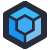Pierre is a contractor who supports a variety of companies helping them manage data-science and analytics applications. Code, in these contexts, is written to answer specific questions, driven more by mathematics than program design. That’s a polite way of saying that the vast majority of the code is unreadable nonsense that no one can explain.

One of Pierre’s clients has decided to migrate to that fancy new thing they’ve been hearing about, “the cloud”, and thus they have to move all of their applications to that cloud. This suite of applications involved a website with a pile of “gadgets” that users could string together to do their own data analysis. And one of those gadgets was quickly thrown together by an intern circa 2007.

Now, no one expects an intern to write good code on their own. They need guidance. They need experience. They absolutely don’t need to be thrown into the deep end to whip up a reusable “gadget” that nobody thinks will ever see production.

So, within that context, the intern did their best. While working for the company, the intern had learned a little bit about GNU Octave, which is what drove most of their analytics. The intern also knew some PHP, from dabbling in web development. Since this particular gadget needed to store some data, the intern brought in the other tool they knew: MySQL.

Thus was born the Intern’s Gadget, which works roughly like this:

• A Cron job launches a PHP script
• The PHP script calls an Octave wrapper script
• The Octave wrapper script loops over a set of configurable parameters, calling a function for each combination
• That function returns MORE Octave code
• Boilerplate is attached to that Octave code by the Octave script pulling content from `start.txt` and `end.txt`
• The Octave wrapper returns the generated code back to the PHP script
• The PHP script writes the generate code to a file
• The PHP script executes the file, collects the results, and stores them in MySQL

The generate file contains one function, called simply `fn`, which is several thousand lines long. Among other things, it contains a chain of nested while loops like:

``````while P(c)>tol | i(1)<=fl(1) | -l(1)+(i(1)+1)*ll(1)-lf(i(1)+1)-log(i(1)-l(1))>tol,
while P(c)>tol | i(2)<=fl(2),
while P(c)>tol | i(3)<=fl(3),
while P(c)>tol | i(4)<=fl(4),
while P(c)>tol | i(5)<=fl(5),
while P(c)>tol | i(6)<=fl(6),
while P(c)>tol | i(7)<=fl(7),
while P(c)>tol | i(8)<=fl(8),
while P(c)>tol | i(9)<=fl(9),
while P(c)>tol | i(10)<=fl(10),
while P(c)>tol | i(11)<=fl(11),
c=c+1;
i(11)=i(11)+1;
k=sum(i);
P(c)=sum(log(XY-k+1:XY))+(XY-k)*lp0+sum(i.*lp)-sum(lf(i+1));
W(c)=sum(i.*w);
end
c=c+1;
i(10)=i(10)+1;
i(11:11)=0;
k=sum(i);
P(c)=sum(log(XY-k+1:XY))+(XY-k)*lp0+sum(i.*lp)-sum(lf(i+1));
W(c)=sum(i.*w);
end
c=c+1;
i(9)=i(9)+1;
i(10:11)=0;
k=sum(i);
P(c)=sum(log(XY-k+1:XY))+(XY-k)*lp0+sum(i.*lp)-sum(lf(i+1));
W(c)=sum(i.*w);
end
c=c+1;
i(8)=i(8)+1;
i(9:11)=0;
k=sum(i);
P(c)=sum(log(XY-k+1:XY))+(XY-k)*lp0+sum(i.*lp)-sum(lf(i+1));
W(c)=sum(i.*w);
end
c=c+1;
i(7)=i(7)+1;
i(8:11)=0;
k=sum(i);
P(c)=sum(log(XY-k+1:XY))+(XY-k)*lp0+sum(i.*lp)-sum(lf(i+1));
W(c)=sum(i.*w);
end
c=c+1;
i(6)=i(6)+1;
i(7:11)=0;
k=sum(i);
P(c)=sum(log(XY-k+1:XY))+(XY-k)*lp0+sum(i.*lp)-sum(lf(i+1));
W(c)=sum(i.*w);
end
c=c+1;
i(5)=i(5)+1;
i(6:11)=0;
k=sum(i);
P(c)=sum(log(XY-k+1:XY))+(XY-k)*lp0+sum(i.*lp)-sum(lf(i+1));
W(c)=sum(i.*w);
end
c=c+1;
i(4)=i(4)+1;
i(5:11)=0;
k=sum(i);
P(c)=sum(log(XY-k+1:XY))+(XY-k)*lp0+sum(i.*lp)-sum(lf(i+1));
W(c)=sum(i.*w);
end
c=c+1;
i(3)=i(3)+1;
i(4:11)=0;
k=sum(i);
P(c)=sum(log(XY-k+1:XY))+(XY-k)*lp0+sum(i.*lp)-sum(lf(i+1));
W(c)=sum(i.*w);
end
c=c+1;
i(2)=i(2)+1;
i(3:11)=0;
k=sum(i);
P(c)=sum(log(XY-k+1:XY))+(XY-k)*lp0+sum(i.*lp)-sum(lf(i+1));
W(c)=sum(i.*w);
end
c=c+1;
i(1)=i(1)+1;
i(2:10)=0;
k=sum(i);
P(c)=sum(log(XY-k+1:XY))+(XY-k)*lp0+sum(i.*lp)-sum(lf(i+1));
W(c)=sum(i.*w);
end``````

There’s no documentation, not in the PHP. Not in the Octave wrapper. Certainly not in the generated code. The one letter variable and function names are pretty standard, all the way through, in both the PHP and the Octave. The intern is long gone, and nobody has touched the code in that time. No one is entirely sure exactly what the gadget does, but users use it and have expectations about how it should work. So when Pierre was tasked with migrating this to the cloud, he did the best he could: they spun up a server, installed Octave and PHP, copied over the scripts, setup the Cron jobs, and pushed the button.

The code was written in 2007, and hadn’t broken once in that time. And once the Cron job fired… it kept right on working. As Pierre says: “It hasn’t broken in 13 years so there’s no need to fix it now. Not that we could.”

The real WTF, then, isn’t the Rube Goldbergian process. It isn’t the cloud transition. It isn’t even handing an important business task to an intern. The real WTF is a statement of awe, because our anonymous intern delivered a piece of software which has been running, error free, for 13 years and delivers real value to the end users, so much so that it had to get translated to an entirely new environment. And it just keeps humming along.

There are people who have spent their entire career in this industry that can’t claim to have accomplished what this intern did, so congratulations, anonymous intern.[Advertisement] ProGet supports your applications, Docker containers, and third-party packages, allowing you to enforce quality standards across all components. Download and see how!